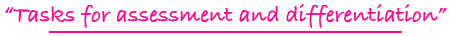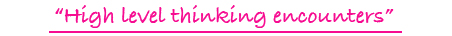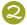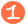•••Tiling Task Card Packets include 20 unique task cards on card stock,  3 backdrop cards , tracking sheets, suggestions and answer sheets.  These are contained in a printed manila envelope for convenient storing.  A set of number tiles 0 - 9 is  needed for each task card

5 - 8

FOUR WAY RELATIONSHIP TILES

completing if...then...relationship statements to find the cost of four items; enrichment problems requiring reasoning and perseverance

Challenge Level:\$15.00

4 - 8

FRACTION TILES DIGITAL

experimenting with number tiles to form common fractions; example: fractions equivalent to 3/4

Challenge Level:DIGITAL FORMAT\$10.00

4 - 8

FRACTION TILES

experimenting with number tiles to form common fractions; example: fractions equivalent to 3/4

Challenge Level:PDF FORMAT
(You will receive both color and black and white files for printing.)\$10.00

4 - 8

FRACTIONAL PART TILES

finding fractional parts of whole numbers; examples: 1/3 of 39; less than 1/4 of 100

Challenge Level:\$15.00

4 - 8

FRACTIONAL PART TILES DIGITAL

finding fractional parts of whole numbers; examples: 1/3 of 39; less than 1/4 of 100

Challenge Level:DIGITAL FORMAT\$10.00

4 - 8

FRACTIONAL PART TILES

finding fractional parts of whole numbers; examples: 1/3 of 39; less than 1/4 of 100

Challenge Level:PDF FORMAT
(You will receive both color and black and white files for printing.)\$10.00

3 - 6

FUNCTION TILES I

figuring and applying rules for functions by looking at input and output numbers: addition, subtraction, multiplication, & division

Challenge Level:\$15.00

5 - 8

FUNCTION TILES II

figuring and applying rules for functions by looking at input and output numbers: functions include 2 operations such as x2 + 1, squaring, square roots

Challenge Level:\$15.00

5 - 8

F(X) TILES

finding x and y in T table fashion when given a function of x, such as f(x) = 3x

Challenge Level:\$15.00

5 - 8

F(X) TILES DIGITAL

finding x and y in T table fashion when given a function of x, such as f(x) = 3x

Challenge Level:DIGITAL FORMAT\$10.00

5 - 8

F(X) TILES

finding x and y in T table fashion when given a function of x, such as f(x) = 3x

Challenge Level:PDF FORMAT
(You will receive both color and black and white files for printing.)\$10.00

1 - 3

GRAPH TILES

forming addition and subtraction problems from data gathered from reading a picture graph

Challenge Level:\$15.00

1 - 3

GRAPH TILES DIGITAL

forming addition and subtraction problems from data gathered from reading a picture graph

Challenge Level:DIGITAL FORMAT\$10.00

1 - 3

GRAPH TILES

forming addition and subtraction problems from data gathered from reading a picture graph

Challenge Level:PDF FORMAT
(You will receive both color and black and white files for printing.)\$10.00

1 - 3

GREATER, EQUAL, LESS THAN TILES DIGITAL

comparing two numbers using the greater than and less than symbols as well as the equal sign

Challenge Level:DIGITAL FORMAT\$10.00

1 - 3

GREATER, EQUAL, LESS THAN TILES

comparing two numbers using the greater than and less than symbols as well as the equal sign

Challenge Level:PDF FORMAT
(You will receive both color and black and white files for printing.)\$10.00

2 - 4

GRID TILES DIGITAL

forming an addition problem, a multiplication problem, and a “half of” problem from pictorial images

Challenge Level:DIGITAL FORMAT\$10.00

2 - 4

GRID TILES

forming an addition problem, a multiplication problem, and a “half of” problem from pictorial images

Challenge Level:PDF FORMAT
(You will receive both color and black and white files for printing.)\$10.00

1 - 3

HIP! HIP! CONCEPT TILES

dealing with before, after, between, more, less, larger, smaller, greater than, etc.

Challenge Level:\$15.00

K - 1

HOW MANY? TILES DIGITAL

counting to 10 and seeing the plus sign along with pictured objects to derive numbers to 10

Challenge Level:DIGITAL FORMAT